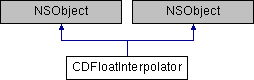cocos2d-x  3.0-rc0
CDFloatInterpolator Class Reference

`#import <CocosDenshion.h>`

Inheritance diagram for CDFloatInterpolator:## Instance Methods

(float) - interpolate:
Return a value between min and max based on t which represents fractional progress where 0 is the start and 1 is the end. More...

(id) - init:startVal:endVal:
var - init:startVal:endVal:
local - init:startVal:endVal:
(float) - interpolate:
Return a value between min and max based on t which represents fractional progress where 0 is the start and 1 is the end. More...

(id) - init:startVal:endVal:

float lastValue

## Properties

float start
float end
tCDInterpolationType interpolationType

## Method Documentation

 - (id) init: (tCDInterpolationType) type startVal: (float) startVal endVal: (float) endVal
 - (id) init: var type startVal: var startVal endVal: var endVal
 - (id) init: local type startVal: local startVal endVal: local endVal
 - (id) init: (tCDInterpolationType) type startVal: (float) startVal endVal: (float) endVal
 - (id) init: var type startVal: var startVal endVal: var endVal
 - (id) init: local type startVal: local startVal endVal: local endVal
 - (float) interpolate: (float) t

Return a value between min and max based on t which represents fractional progress where 0 is the start and 1 is the end.

 - (float) interpolate: var t

Return a value between min and max based on t which represents fractional progress where 0 is the start and 1 is the end.

 - (float) interpolate: local t

Return a value between min and max based on t which represents fractional progress where 0 is the start and 1 is the end.

 - (float) interpolate: (float) t

Return a value between min and max based on t which represents fractional progress where 0 is the start and 1 is the end.

 - (float) interpolate: var t

Return a value between min and max based on t which represents fractional progress where 0 is the start and 1 is the end.

 - (float) interpolate: local t

Return a value between min and max based on t which represents fractional progress where 0 is the start and 1 is the end.

## Member Data Documentation

 - (float) lastValue
protected
 var lastValue
protected
 local lastValue
protected

## Property Documentation

 - (float) end
 var end
 local end
 - (tCDInterpolationType) interpolationType